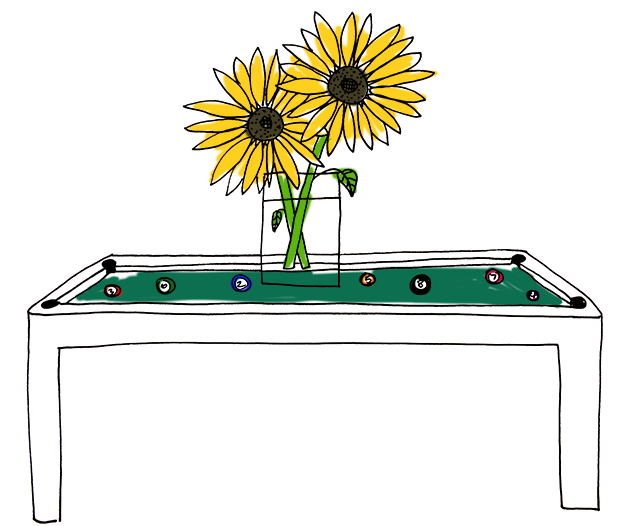# Diverging pathsThe error in the previous problem doesn't grow with time. This is a hallmark of a nonchaotic system. We can characterize this more formally by saying that nearby paths don't diverge. All the ball trajectories that started out near the origin stayed together over time.

In a chaotic system, on the other hand, nearby paths diverge, even if they are infinitesimally nearby. In such a system the time evolution becomes unpredictable, as you would need to know the initial conditions of the system to infinite accuracy. This is chaos!

It is possible to turn a billiard table into a chaotic billiard table, or one where the trajectory of the ball becomes unpredictable over long times if there is any error. To do this we can put a shape in the middle of the table off which the ball will bounce.

Suppose we start the ball at the bottom of the table at (0,-2) with an error $\epsilon$ in the $x$-component of its position. The initial velocity is (0,1). Which shape in the middle will cause the motion of the ball to become chaotic?

×

Problem Loading...

Note Loading...

Set Loading...